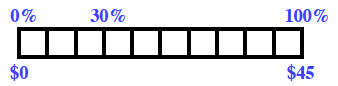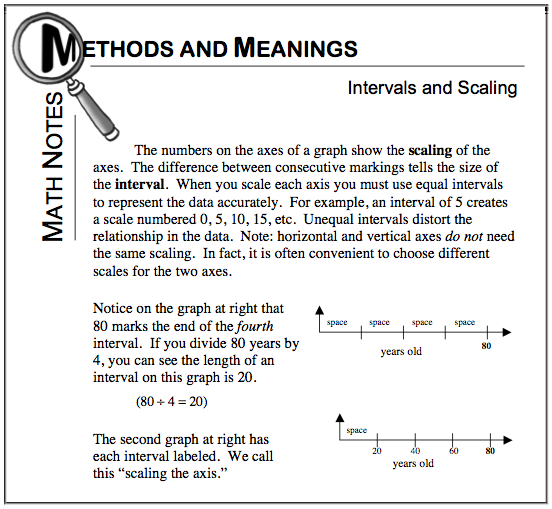Home > ACC7 > Chapter cc27 > Lesson cc27.1.5 > Problem7-61

7-61.

Tires R Us is having a $30\%$-off sale. Find the savings on a tire that regularly costs $\45$. Homework Help ✎

Your percent ruler might look something like this:Using the Math Notes box below as a reference, can you scale the rest of the ruler in order to find the $30\%$ equivalent in dollars? It may help to first find the value of $10\%$.

$30\%$ of $\45$ is $\13.50$. Remember to show your work!